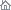Home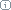About Us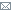ContactBlogWhat's NewProductsBuy NowDownloadsForumGeneXproTools Online Guide Learn how to use the 5 modeling platforms of GeneXproTools with the Online GuideLast update: February 19, 2014Derived Variables

Derived variables (also called new features), as the name suggests, are input variables derived from the original variables. They can range from simple transformations such as taking the log of input variables to complex functions involving all input variables. In GeneXproTools derived variables are created and managed in the Static UDFs Tab in the Functions Panel. In Karva notation they are represented as UDF0, UDF1,…, UDFn.

Derived variables are programmed in the UDF Editor and must be coded in JavaScript. GeneXproTools allows you to import the code of derived variables from other gep files, giving you easy access to your favorite UDFs.

The output of all derived variables created in GeneXproTools can be analyzed and visualized in the Data Panel. You can analyze their scatter plots against any other variable, plot their histograms, analyze different line charts, compute and visualize summary statistics, and several other analyses.

In modeling terms, derived variables are handled by the learning algorithms of GeneXproTools in exactly the same way as the original variables, that is, during model evolution GeneXproTools uses both variable types interchangeably, combining them in different ways and integrating them in different model structures or modules. The JavaScript code below is a regression model that combines 3 derived variables and 7 of the original variables.
```//------------------------------------------------------------------
// Regression model generated by GeneXproTools 5.0 on 5/20/2013 10:00:10 PM
// GEP File: D:\GeneXproTools\Version5.0\OnlineGuide\ConcreteStrength-UDFs_01a.gep
// Training Records:  687
// Validation Records:   343
// Fitness Function:  Positive Correl
// Training Fitness:  914.425772796843
// Training R-square: 0.836174493955104
// Validation Fitness:   919.274374939755
// Validation R-square:  0.845065376420878
//------------------------------------------------------------------

var terminals = new Array();

function gepModel(d)
{
var G1C2 = -0.821369315164647;
var G1C0 = -2.88029035889767;
var G3C2 = 3.40349775688955;
var G4C1 = 2.88776641366202;
var G5C5 = 11.260200627471;
var G5C3 = 5.7177285881222;
var G5C9 = -10.2894261546678;
var G5C2 = 4.06598101748711;
var G6C3 = 6.55475921536912;
var G6C0 = 13.2330739691954;

terminals = d;

var vTemp = 0.0;

vTemp = ((UDF1()/Math.pow(Math.atan(Math.min(((G1C0+d)/2.0),G1C2)),2))-d);
vTemp += (1-UDF3());
vTemp += ((gep3Rt(d)+Math.min(((d+d)/2.0),(G3C2+d)))+UDF2());
vTemp += (UDF1()-gep3Rt(Math.pow(((Math.max(G4C1,d)-d)+(G4C1-d)),2)));
vTemp += Math.min((((((d+G5C2)+d)/2.0)+(1-d))/2.0),((G5C5*d)-(G5C3*G5C9)));
vTemp += (Math.min((((d+d)/2.0)+G6C3),(G6C0*d))-(((d+d)/2.0)+(d+d)));

return vTemp;
}

function gep3Rt(x)
{
return x < 0.0 ? -Math.pow(-x,(1.0/3.0)) : Math.pow(x,(1.0/3.0));
}

function UDF1()
{
// Average Model
var nVariables = terminals.length;
var sum = 0.0;
var averageModel = 0.0;
for (var nV=0; nV < nVariables; nV++)
{
sum += terminals[nV];
}
averageModel = sum / nVariables;

return averageModel;
}

function UDF2()
{
// Sum
var nVariables = terminals.length;
var sum = 0.0;
for (var nV=0; nV < nVariables; nV++)
{
sum += terminals[nV];
}

return sum;
}

function UDF3()
{
// Max Model
var nVariables = terminals.length;
var maxModel = terminals;
for (var nV=1; nV < nVariables; nV++)
{
if (terminals[nV] > maxModel)
maxModel = terminals[nV];
}

return maxModel;
}
```

GeneXproTools evaluates the variable importance of all the variables (original and derived) in a model. The variable importance is also shown in the Statistics Charts in the Data Panel.

Related Tutorials:

Related Videos:

 Leave Feedback Please enter the number below using the combo boxes before sending your feedback. 3 8 4 0 1 2 3 4 5 6 7 8 9   0 1 2 3 4 5 6 7 8 9   0 1 2 3 4 5 6 7 8 9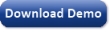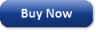Time Limited Trial

Released February 19, 2014

Last update: 5.0.3883New Entries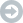Subscribe to the GEP-List

 3 8 4 0 1 2 3 4 5 6 7 8 9   0 1 2 3 4 5 6 7 8 9   0 1 2 3 4 5 6 7 8 9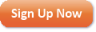Home | What's New | Products | Buy Now | Downloads | Quick Tour | Support | Contact Us | About Gepsoft | Sign UpForum | Blog | Videos | Tutorials | Server Knowledge Base | Logistic Regression Guide | Terms of Use | Privacy & Cookies